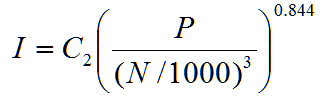H20Calc (Retired)

# Inertia of Pumps and Motors from Innovyze H2OCalc – Idea for SWMM6

Inertia of Pumps and Motors

The combined inertia of pumps and motors driving them, including the connecting shafts and couplings, is required for transient analysis associated with the starting and stopping of pumps. The equations provided below are intended to be used as an initial guide to the inertia values that may be used as a reasonable first approximation, when more accurate data is not available. The total inertia for the pump/motor unit is the sum of both pump and motor inertias. The following inertia calculations are based on Thorley (2004).

Pump Inertias

From the linear regression analysis of 300 pump inertia data, two equations were developed for predicting the inertia I of pump impellers, including the entrained water and the shaft on which the impeller is mounted. The first equation represents the upper set of the data, and applies to single- and double-entry impellers, single and multistage, and horizontal and vertical, spindle machines.where I = pump inertia (kg m2, lb ft2)

C1 = coefficients (0.03768, 0.6674)

P = power (kW, hp)

N = pump speed (rev/min)

The second equation is for lower set of the data and represents relatively small, single-entry, radial flow impellers of lightweight design. This is applied to relatively small pumps of lightweight design.where C2 = coefficients (0.03407 in SI, 0.6244 in English)

Motor Inertias

Similar to pump inertia, linear regression of the motor inertia data yields the following equations.where C3 = coefficients (0.0043 in SI, 0.0648 in English).